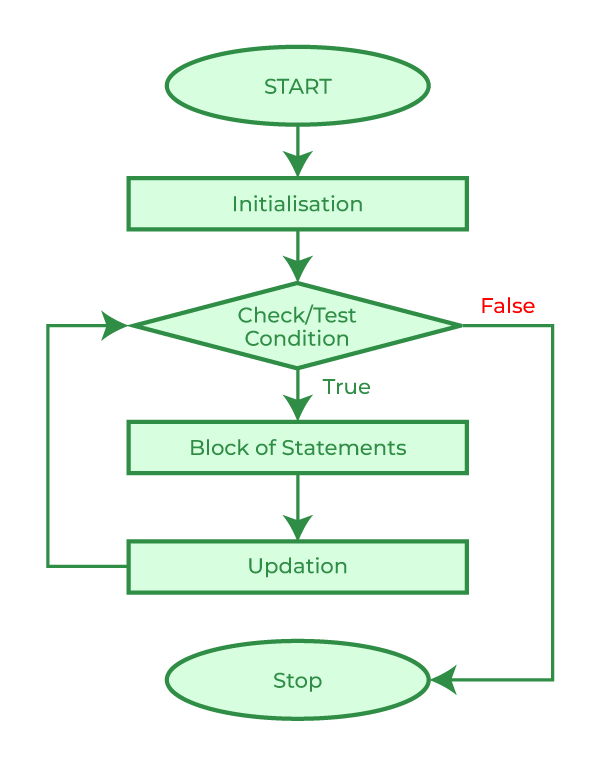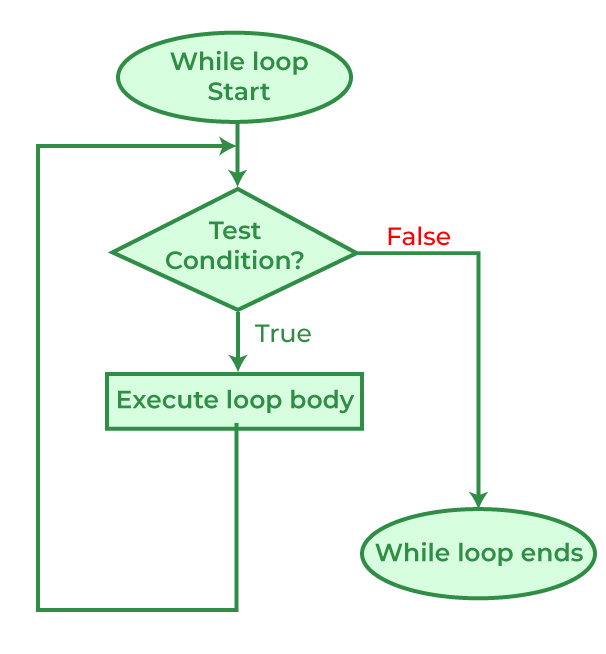Open In App

# Difference between for and while loop in C, C++, Java

In C, C++, and Java, both for loop and while loop is used to repetitively execute a set of statements a specific number of times. However, there are differences in their declaration and control flow. Let’s understand the basic differences between a for loop and a while loop.

## for Loop

A for loop provides a concise way of writing the loop structure. Unlike a while loop, a for loop declaration consumes the initialization, condition, and increment/decrement in one line thereby providing a shorter, easy-to-debug structure of looping.

### Syntax

```for (initialization condition; testing condition; increment/decrement)
{
// statement(s)
}```

## Flowchart of for Loop## C

 `// C Program to illustrate the use of for loop``#include `` ` `int` `main()``{``    ``// loop variable``    ``int` `i = 0;`` ` `    ``// for loop that prints "GFG" 5 times``    ``for` `(i = 5; i < 10; i++) {``        ``printf``(``"GFG\n"``);``    ``}`` ` `    ``return` `0;``}`

## C++

 `// CPP program to demonstrate``// for loop`` ` `#include ``using` `namespace` `std;`` ` `int` `main()``{``    ``// Initialize variable 'i' with a value of 0``    ``int` `i = 0;`` ` `    ``// Iterate using a for loop from 5 to 9 (inclusive)``    ``for` `(i = 5; i < 10; i++) {`` ` `        ``// Print "GFG" on each iteration``        ``cout << ``"GFG\n"``;``    ``}`` ` `    ``return` `0;``}`

## Java

 `// Java program to illustrate the use of for loop``import` `java.io.*;`` ` `class` `GFG {``    ``public` `static` `void` `main(String[] args)``    ``{``        ``// loop variable``        ``int` `i = ``0``;`` ` `        ``// for loop``        ``for` `(i = ``5``; i < ``10``; i++) {``            ``System.out.println(``"GfG"``);``        ``}``    ``}``}`

Output

```GFG
GFG
GFG
GFG
GFG
```

## C

 `// C program to demonstrate``// infinite for loop``#include `` ` `int` `main()``{`` ` `    ``// Infinite loop using a for loop with no condition``    ``// specified The absence of a condition means the loop``    ``// will continue indefinitely until it is explicitly``    ``// interrupted or terminated.``    ``for` `(;;) {``        ``printf``(``"GFG\n"``);``    ``}`` ` `    ``return` `0;``}`

## C++

 `// C++ program to demonstrate``// infinite for loop``#include ``using` `namespace` `std;`` ` `int` `main()``{``    ``// Infinite loop using a for loop with no condition``    ``// specified The absence of a condition means the loop``    ``// will continue indefinitely until it is explicitly``    ``// interrupted or terminated.``    ``for` `(;;) {`` ` `        ``// Print "GFG" on each iteration``        ``cout << ``"GFG\n"``;``    ``}`` ` `    ``return` `0;``}`

## Java

 `// Java program to illustrate the use of for loop``import` `java.io.*;`` ` `class` `GFG {``    ``public` `static` `void` `main(String[] args)``    ``{``        ``// infinite for loop``        ``for` `(;;) {``            ``System.out.println(``"GFG!"``);``        ``}``    ``}``}`

Output

```GFG
GFG
GFG
...
...
...
{truncated}```

## while Loop

A while loop is a control flow statement that allows code to be executed repeatedly based on a given Boolean condition. The while loop can be thought of as a repeating if statement.

### Syntax

```while (boolean condition)
{
// loop statements...
}```

## Flowchart of while Loop## C

 `// C program to demonstrate while loop``#include `` ` `int` `main()``{``    ``// loop variable definition``    ``int` `i = 5;`` ` `    ``// while loop that prints "GFG" 5 times``    ``while` `(i < 10) {``        ``printf``(``"GFG\n"``);``        ``i++;``    ``}`` ` `    ``return` `0;``}`

## C++

 `// C++ program to demonstrate``// while loop``#include ``using` `namespace` `std;`` ` `int` `main()``{``    ``// Initialize variable 'i' with a value of 5``    ``int` `i = 5;`` ` `    ``// Execute the loop as long as 'i' is less than 10``    ``while` `(i < 10) {`` ` `        ``// Increment 'i' by 1 on each iteration``        ``i++;`` ` `        ``// Print "GFG" on each iteration``        ``cout << ``"GFG\n"``;``    ``}`` ` `    ``return` `0;``}`

## Java

 `import` `java.io.*;`` ` `class` `GFG {``    ``public` `static` `void` `main(String[] args)``    ``{`` ` `        ``int` `i = ``5``;`` ` `        ``while` `(i < ``10``) {``            ``i++;``            ``System.out.println(``"GfG"``);``        ``}``    ``}``}`

Output

```GFG
GFG
GFG
GFG
GFG
```

## C

 `// C program to domonstrate``// infinite while loop``#include `` ` `int` `main()``{``    ``// Condition is always true which results in infinite``    ``// loop``    ``while` `(1)``        ``printf``(``"GFG\n"``);`` ` `    ``return` `0;``}`

## C++

 `// C++ program to domonstrate``// infinite while loop``#include ``using` `namespace` `std;`` ` `int` `main()``{``    ``// Condition is always true which results in infinite``    ``// loop``    ``while` `(1)``        ``cout << ``"GFG\n"``;`` ` `    ``return` `0;``}`

## Java

 `// C++ program to domonstrate``// infinite while loop``import` `java.io.*;`` ` `class` `GFG {``    ``public` `static` `void` `main(String[] args)``    ``{``        ``// loop variable``        ``int` `i = ``5``;`` ` `        ``// while loop``        ``while` `(i < ``10``) {``            ``System.out.println(``"GFG\n"``);``        ``}``    ``}``}`

Output

```GFG
GFG
GFG
...
...
...
{truncated}```

## Difference Between for Loop and while Loop

The major differences between for loop and while loop in C, C++ and Java are as follows: